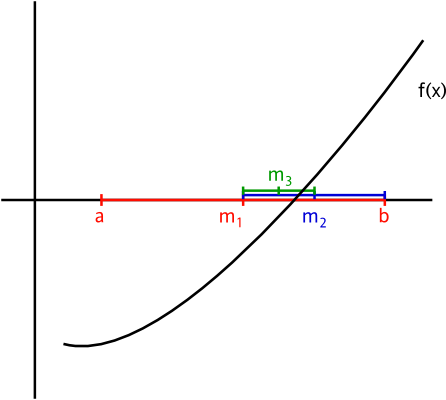# C Program for Bisection Method

Bisection Method, is a Numerical Method, used for finding a root of an equation.
The method is based upon bisecting an interval that brackets(contains) the root repeatedly, until the approximate root is found.In this post I will show you how to write a C Program in various ways to find the root of an equation using the Bisection Method.

The following is a simple version of the program that finds the root, and tabulates the different values at each iteration. Just like any other numerical method bisection method is also an iterative method, so it is advised to tabulate values at each iteration.

### PROGRAM(Simple Version):

```/******************************
******BISECTION  METHOD*******
******************************
2017 (c) Manas Sharma - https://bragitoff.com
*******************************/
#include<stdio.h>
#include<math.h>

/*Function whose root is to be determined*/
double f(double x){
return 3*x+sin(x)-exp(x);
}

int main(){
double a,b,c,eps;
int maxSteps;
a:printf("Enter the initial guess a:\n");
scanf("%lf",&a);
printf("Enter the initial guess b:\n");
scanf("%lf",&b);
printf("Enter the desired accuracy:\n");
scanf("%lf",&eps);
printf("Enter the max. number of steps:\n");
scanf("%d",&maxSteps);
if(f(a)*f(b)<=0){
int iter=1;
/*Bisection Method begins that tabulates the various values at each iteration*/
printf("____________________________________________________________________________________\n");
printf("iter\ta\t\tb\t\tc\t\tf(c)\t\t|a-b|\n");
printf("____________________________________________________________________________________\n");
do{
c=(a+b)/2;
printf("%d.\t%lf\t%lf\t%lf\t%lf\t%lf\n",iter,a,b,c,f(c),fabs(a-b));
if(f(a)*f(c)>0){
a=c;
}
else if(f(a)*f(c)<0){
b=c;
}
iter++;

}while(fabs(a-b)>=eps&&iter<=maxSteps);
printf("_______________________________________________________________________________________\n\nOne of the roots of the given equation is:\n\n%lf\n\n\n",c);
}
else{
printf("\nSorry! the root doesn't exist in the given interval.\nPlease enter a different set of guesses.\n");
goto a;
}

}
```

The better version of the above code uses a function called ‘bisection’ to perform the bisection task and return the root.
However, this function won’t tabulate the values at each iteration.
So in the following program I have also provided another function called ‘printBisection’ that would return the root as well as print the various values at each iteration.

### PROGRAM(Better Version):

```/*****************************************
************BISECTION METHOD*************
2017 (c) Manas Sharma - https://bragitoff.com
****************************************/
#include<stdio.h>
#include<math.h>
/*Function whose root is to be determined*/
double f(double x){
return x*x-4;
}
/*Function definition for bisection procedure[Returns the root if found or 999 for failure]*/
double bisection(double f(double x),double a, double b, double eps, int maxSteps){
double c;
if(f(a)*f(b)<=0){
int iter=1;
/*Bisection Method begins that tabulates the various values at each iteration*/
do{
c=(a+b)/2;
if(f(a)*f(c)>0){
a=c;
}
else if(f(a)*f(c)<0){
b=c;
}
iter++;

}while(fabs(a-b)>=eps&&iter<=maxSteps);
return c;
}
else{
return 999;
}
}
/*The following function performs the bisection procedure and also prints the values of various variables at each iteration */
double printBisection(double f(double x),double a, double b, double eps, int  maxSteps){
double c;
if(f(a)*f(b)<=0){
int iter=1;
/*Bisection Method begins that tabulates the various values at each iteration*/
printf("____________________________________________________________________________________\n");
printf("iter\ta\t\tb\t\tc\t\tf(c)\t\t|a-b|\n");
printf("____________________________________________________________________________________\n");
do{
c=(a+b)/2;
printf("%d.\t%lf\t%lf\t%lf\t%lf\t%lf\n",iter,a,b,c,f(c),fabs(a-b));
if(f(a)*f(c)>0){
a=c;
}
else if(f(a)*f(c)<0){
b=c;
}
iter++;

}while(fabs(a-b)>=eps&&iter<=maxSteps);
printf("_______________________________________________________________________________________\n\nOne of the roots of the given equation is:\n\n%lf\n\n\n",c);
}
else{
printf("\nSorry! the root doesn't exist in the given interval.\nPlease enter a different set of guesses.\n");
}
}
main(){
double a,b,eps;
int maxSteps;
printf("Enter the initial guess a:\n");
scanf("%lf",&a);
printf("\nEnter the inital guess b:\n");
scanf("%lf",&b);
printf("\nEnter the desired accuracy:\n");
scanf("%lf",&eps);
printf("Enter the max. number of steps:\n");
scanf("%d",&maxSteps);
printBisection(f,a,b,eps,maxSteps);
}
```

### Related Posts:[wpedon id="7041" align="center"]